# The MDC Procedure

### HEV and Multinomial Probit: Heteroscedastic Utility Function

When the stochastic components of utility are heteroscedastic and independent, you can model the data by using an HEV or a multinomial probit model. The HEV model assumes that the utility of alternative j for each individual i has heteroscedastic random components,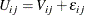where the cumulative distribution function of the Gumbel distributed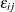is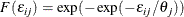Note that the variance ofis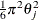. Therefore, the error variance is proportional to the square of the scale parameter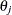. For model identification, at least one of the scale parameters must be normalized to 1. The following SAS statements estimate an HEV model under a unit scale restriction for mode "1" (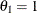):

/*-- hev with gauss-laguerre method --*/
proc mdc data=newdata;
model decision = ttime /
type=hev
nchoice=3
hev=(unitscale=1, integrate=laguerre)
covest=hess;
id pid;
run;


The results of computation are presented in Figure 18.14 and Figure 18.15.

Figure 18.14: HEV Estimation Summary

The MDC Procedure

Heteroscedastic Extreme Value Model Estimates

Model Fit Summary
Dependent Variable decision
Number of Observations 50
Number of Cases 150
Log Likelihood -33.41383
Maximum Absolute Gradient 0.0000218
Number of Iterations 11
Optimization Method Dual Quasi-Newton
AIC 72.82765
Schwarz Criterion 78.56372

Figure 18.15: HEV Parameter Estimates

The MDC Procedure

Heteroscedastic Extreme Value Model Estimates

Parameter Estimates
Parameter DF Estimate Standard
Error
t Value Approx
Pr > |t|
ttime 1 -0.4407 0.1798 -2.45 0.0143
SCALE2 1 0.7765 0.4348 1.79 0.0741
SCALE3 1 0.5753 0.2752 2.09 0.0366

The parameters SCALE2 and SCALE3 in the output correspond to the estimates of the scale parameters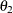and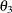, respectively.

Note that the estimate of the HEV model is not always stable because computation of the log-likelihood function requires numerical integration. Bhat (1995) proposed the Gauss-Laguerre method. In general, the log-likelihood function value of HEV should be larger than that of conditional logit because HEV models include the conditional logit as a special case. However, in this example the reverse is true (–33.414 for the HEV model, which is less than –33.321 for the conditional logit model). (See Figure 18.14 and Figure 18.3.) This indicates that the Gauss-Laguerre approximation to the true probability is too coarse. You can see how well the Gauss-Laguerre method works by specifying a unit scale restriction for all modes, as in the following statements, since the HEV model with the unit variance for all modes reduces to the conditional logit model:

/*-- hev with gauss-laguerre and unit scale --*/
proc mdc data=newdata;
model decision = ttime /
type=hev
nchoice=3
hev=(unitscale=1 2 3, integrate=laguerre)
covest=hess;
id pid;
run;


Figure 18.16 shows that the ttime coefficient is not close to that of the conditional logit model.

Figure 18.16: HEV Estimates with All Unit Scale Parameters

The MDC Procedure

Heteroscedastic Extreme Value Model Estimates

Parameter Estimates
Parameter DF Estimate Standard
Error
t Value Approx
Pr > |t|
ttime 1 -0.2926 0.0438 -6.68 <.0001

There is another option of specifying the integration method. The INTEGRATE=HARDY option uses the adaptive Romberg-type integration method. The adaptive integration produces much more accurate probability and log-likelihood function values, but often it is not practical to use this method of analyzing the HEV model because it requires excessive CPU time. The following SAS statements produce the HEV estimates by using the adaptive Romberg-type integration method:

/*-- hev with adaptive integration --*/
proc mdc data=newdata;
model decision = ttime /
type=hev
nchoice=3
hev=(unitscale=1, integrate=hardy)
covest=hess;
id pid;
run;


The results are displayed in Figure 18.17 and Figure 18.18.

Figure 18.17: HEV Estimation Summary Using Alternative Integration Method

The MDC Procedure

Heteroscedastic Extreme Value Model Estimates

Model Fit Summary
Dependent Variable decision
Number of Observations 50
Number of Cases 150
Log Likelihood -33.02598
Maximum Absolute Gradient 0.0001202
Number of Iterations 8
Optimization Method Dual Quasi-Newton
AIC 72.05197
Schwarz Criterion 77.78803

Figure 18.18: HEV Estimates Using Alternative Integration Method

The MDC Procedure

Heteroscedastic Extreme Value Model Estimates

Parameter Estimates
Parameter DF Estimate Standard
Error
t Value Approx
Pr > |t|
ttime 1 -0.4580 0.1861 -2.46 0.0139
SCALE2 1 0.7757 0.4283 1.81 0.0701
SCALE3 1 0.6908 0.3384 2.04 0.0412

With the INTEGRATE=HARDY option, the log-likelihood function value of the HEV model,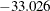, is greater than that of the conditional logit model,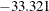. (See Figure 18.17 and Figure 18.3.)

When you impose unit scale restrictions on all choices, as in the following statements, the HEV model gives the same estimates as the conditional logit model. (See Figure 18.19 and Figure 18.6.)

/*-- hev with adaptive integration and unit scale --*/
proc mdc data=newdata;
model decision = ttime /
type=hev
nchoice=3
hev=(unitscale=1 2 3, integrate=hardy)
covest=hess;
id pid;
run;


Figure 18.19: Alternative HEV Estimates with Unit Scale Restrictions

The MDC Procedure

Heteroscedastic Extreme Value Model Estimates

Parameter Estimates
Parameter DF Estimate Standard
Error
t Value Approx
Pr > |t|
ttime 1 -0.3572 0.0776 -4.60 <.0001

For comparison, the following statements estimate a heteroscedastic multinomial probit model by imposing a zero restriction on the correlation parameter,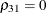. The MDC procedure requires normalization of at least two of the error variances in the multinomial probit model. Also, for identification, the correlation parameters associated with a unit normalized variance are restricted to be zero. When the UNITVARIANCE= option is specified, the zero restriction on correlation coefficients applies to the last choice of the list. In the following statements, the variances of the first and second choices are normalized. The UNITVARIANCE=(1 2) option imposes additional restrictions that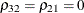. The default for the UNITVARIANCE= option is the last two choices (which would have been equivalent to UNITVARIANCE=(2 3) for this example). The result is presented in Figure 18.20.

The utility function can be defined aswhere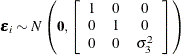/*-- mprobit estimation --*/
proc mdc data=newdata;
model decision = ttime /
type=mprobit
nchoice=3
unitvariance=(1 2)
covest=hess;
id pid;
restrict RHO_31 = 0;
run;


Figure 18.20: Heteroscedastic Multinomial Probit Estimates

The MDC Procedure

Multinomial Probit Estimates

Parameter Estimates
Parameter DF Estimate Standard
Error
t Value Approx
Pr > |t|
Parameter Label
ttime 1 -0.3206 0.0920 -3.49 0.0005
STD_3 1 1.6913 0.6906 2.45 0.0143
RHO_31 0 0 0
Restrict1 1 1.1854 1.5490 0.77 0.4499* Linear EC [ 1 ]

 * Probability computed using beta distribution.

Note that in the output the estimates of standard errors and correlations are denoted by STD_i and RHO_ij, respectively. In this particular case the first two variances (STD_1 and STD_2) are normalized to one, and corresponding correlations (RHO_21 and RHO_32) are set to zero, so they are not listed among parameter estimates.